calculator.org

# Scientific/Engineering Functions

##- Reciprocal

Computes the reciprocal of the number currently displayed.

##- Square Root

Computes the square root of the number currently displayed.

##- Square

Computes the square of the number currently displayed.

##- Logarithm to base 10

Computes the logarithm to base 10 of the number currently displayed.

##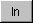- Natural Logarithm

Computes the natural logarithm (logarithm to base e) of the number currently displayed.

##- X to the Power of a

Computes X to the power of a.

Example:
Calculate 5 raised to the power of 6.

56Result: 15625.000000

##- ath Root of X

Computes the ath root of X (i.e. X to the power of the reciprocal of a).

Example:
Calculate the cube root of two:

23Result: 1.259921004989

##- Input Degrees/Hours, Minutes, Seconds (Sexagesimal)

The deg or hour button is used to enter an angle/time in degrees/hours, minutes and seconds in one of the sexagesimal modes (i.e. Angle or Time). The button appears where the rand button appears in Decimal modes.

To enter a number in sexagesimal format, first enter the number of whole degrees or hours, and press degree or hour. The legend changes to minute to indicate that the next number input is the number of minutes. Enter the number of minutes and press minute. The legend changes again, this time to second. Enter the number of seconds.

Arithmetic calculations can be carried out in Angle or Time mode, or the result or number entered can be converted to another format by selecting a different calculator mode. Similarly a Decimal (or other base) number can be converted to sexagesimal format by selecting the Angle or Time mode.

Note that selecting Time or Angle mode automatically selects appropriate properties and units for conversion.

Example 1:
What is the sine of 17 degrees, 14 minutes and 30 seconds?

First click on, select Angle mode in the mode dialog box (or Alt Shift A).

1 7 o 1 4 ' 3 0 "
Result: 17° 14' 30"

Click onto change to Decimal mode or use the Options/Mode page (or Alt Shift D):
Result: 17.2416666666667

sin
Result: 0.2964026699

Example 2:
I started work at 08:30 and finished at 18:54, and I took a 45 minute lunch break. How many hours do I put on my time sheet?

First clickor select Time mode in the Options/Mode page (or Alt Shift T).

1 8 hr 5 4 ' - 8 hr 3 0 ' - hr 4 5 ' =
Result: 9h 39' 0"

To continue the calculation to accumulate hours for a weekly time sheet, use the m+ button. To convert to a decimal number of hours, select Decimal mode (or Alt Shift D).

##- Random Number

Generates a pseudo-random number between 0 and 1.

##- Hyperbolic

This button is used to select hyperbolic functions and inverse hyperbolic functions. Clicking the hyp button causes the legends of the sin, cos and tan to change to sinh, cosh and tanh.

Pressing shift either before or after the hyp causes the inverse hyperbolic functions to be displayed: asinh, acosh and atanh.

Example:
Compute the hyperbolic sin of 1.0

1 hyp sinh
Result: 1.175201193643801

##- Sine

Computes the sine of an angle.

##- Inverse Sine

Computes the arc sine, i.e. the angle for which the sine is the value entered.

##- Cosine

Computes the cosine of an angle.

##- Inverse Cosine

Computes the angle for which the cosine is the value entered.

##- Tangent

Computes the tangent of an angle.

##- Inverse Tangent

Computes the angle for which the tangent is the value entered.

##- Factorial

Computes the factorial of a current number. If the current number displayed is not an integer, the result is the factorial of the integer value of the current number.

##- Pi

Enters the value of pi, the ratio of the circumference of a circle to it's diameter, in the display.

##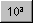- Inverse Logarithm

Computes 10 raised to the power of the current number, giving the inverse logarithm.

##- Inverse Natural Logarithm

Computes the value of e raised to the power of the current number (exponential function or inverse of natural logarithm).

##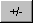- Sign

Changes the sign of the number currently displayed.

##- Backspace

Use this button to remove the most recently entered numbers, digit by digit.

To remove the whole of an entry, use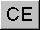(Clear entry).

##- Left Parenthesis

Theand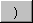buttons are used to enforce the order of evaluation of an expression. For example, the expression 1 + 2 X 3 would be evaluated as 9 if operator precedence is determined by the order of entry. This is a different answer to the same expression evaluated using algebraic precedence or in a programming language such as BASIC, C or FORTRAN, which gives multiplication and division precedence over addition and subtraction.

To force the calculator to evaluate a particular expression first, use parentheses (brackets). Evaluating 1 + (2 X 3) always gives the result 7. The current level of parentheses is shown in the button legend.

See ).

##- Right Parenthesis

Closes a level of parentheses. The current level of parentheses is shown in the button legend.

See (.